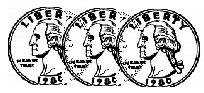KCC5
 Name:    KCC5

Multiple Choice
Identify the choice that best completes the statement or answers the question.

1.

Tell how many footballs there are.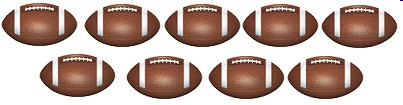a. 12 c. 9 b. 5 d. 7

2.

Tell how many apples there are.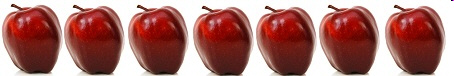a. 12 c. 9 b. 5 d. 7

3.

Which shows 10?
 a.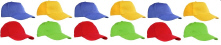c.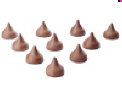b.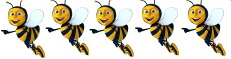d.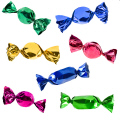4.

Which shows 8?
 a.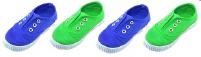c.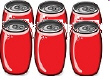b.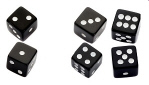d.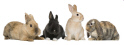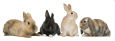5.

Count how many blocks there are.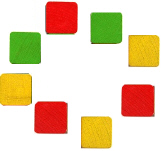a. 8 c. 9 b. 7 d. 10

Completion
Complete each statement.

6.

Tell how many.7.

How many cones are there?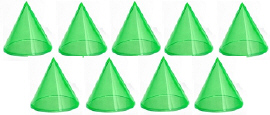8.

How many coins are there?